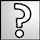Trycera_Paul: Goodevenin to u to my love abhirajABHIRAJ: Good Evening Trycera Paul my sweetheart my love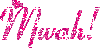ABHIRAJ: Good morning my love my sweetheart Trycera Paul 🫂win 10.000 +s for correct ans
(23:48) Fri, 19 May 17Turn the 6 into 0 by moving the stick in the middle towards the right. This makes the equation,
0 + 4 = 4
(18:48) Sun, 21 May 17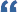Quote: haris: single on 2wap, Atleast?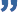I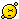now she will not.
(16:49) Sun, 21 May 17Quote: _Ankit_: 6+4=4. 6is divided by 3 =2 and 4is divided by 2 =2 and 2+2=4This is why i hate answering to math puzzle..
(15:08) Sun, 21 May 17Quote: _Ankit_: 6+4=4. 6is divided by 3 =2 and 4is divided by 2 =2 and 2+2=4Move only one match
(13:15) Sun, 21 May 17Quote: _Ankit_: 6+4=4. 6is divided by 3 =2 and 4is divided by 2 =2 and 2+2=4Complicated sperm
(05:09) Sun, 21 May 176+4=4. 6is divided by 3 =2 and 4is divided by 2 =2 and 2+2=4
(03:39) Sun, 21 May 17Quote: merly: Not singlesingle on 2wap, Atleast?
(13:09) Sat, 20 May 17Not single
(11:58) Sat, 20 May 17Quote: merly: 8-4=4 ? I remove one stick on the plus sign n put it in 6 to bcome 8are you single?
(11:33) Sat, 20 May 178-4=4 ? I remove one stick on the plus sign n put it in 6 to bcome 8
(05:24) Sat, 20 May 170 + 4= 4
(03:04) Sat, 20 May 17Krack are you working for jaqui?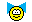(02:26) Sat, 20 May 17I add 6 to 44 =50
(01:29) Sat, 20 May 17Quote: krack: Idk..am poor in Maths .sad.its easy swty
(23:55) Fri, 19 May 17